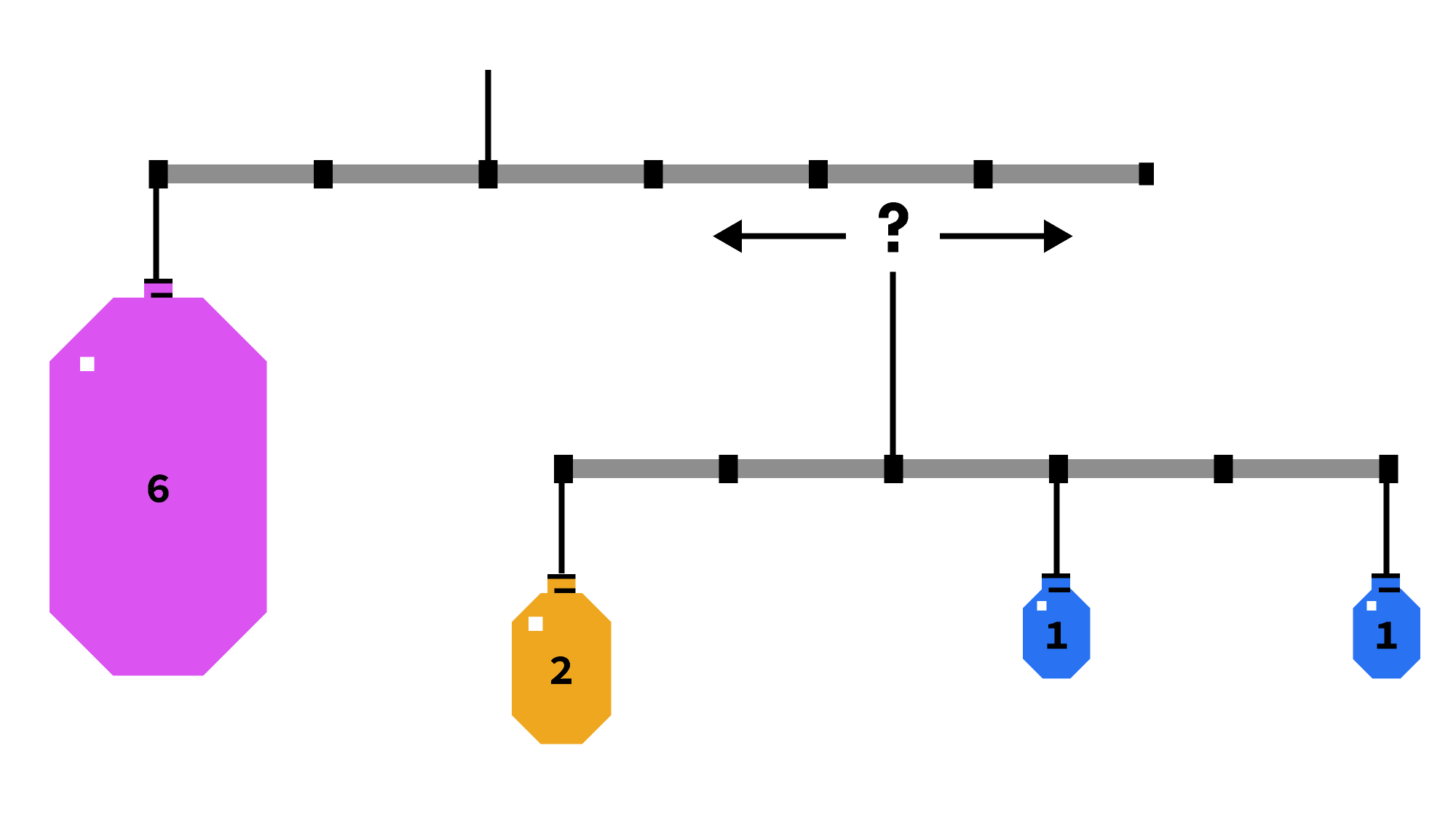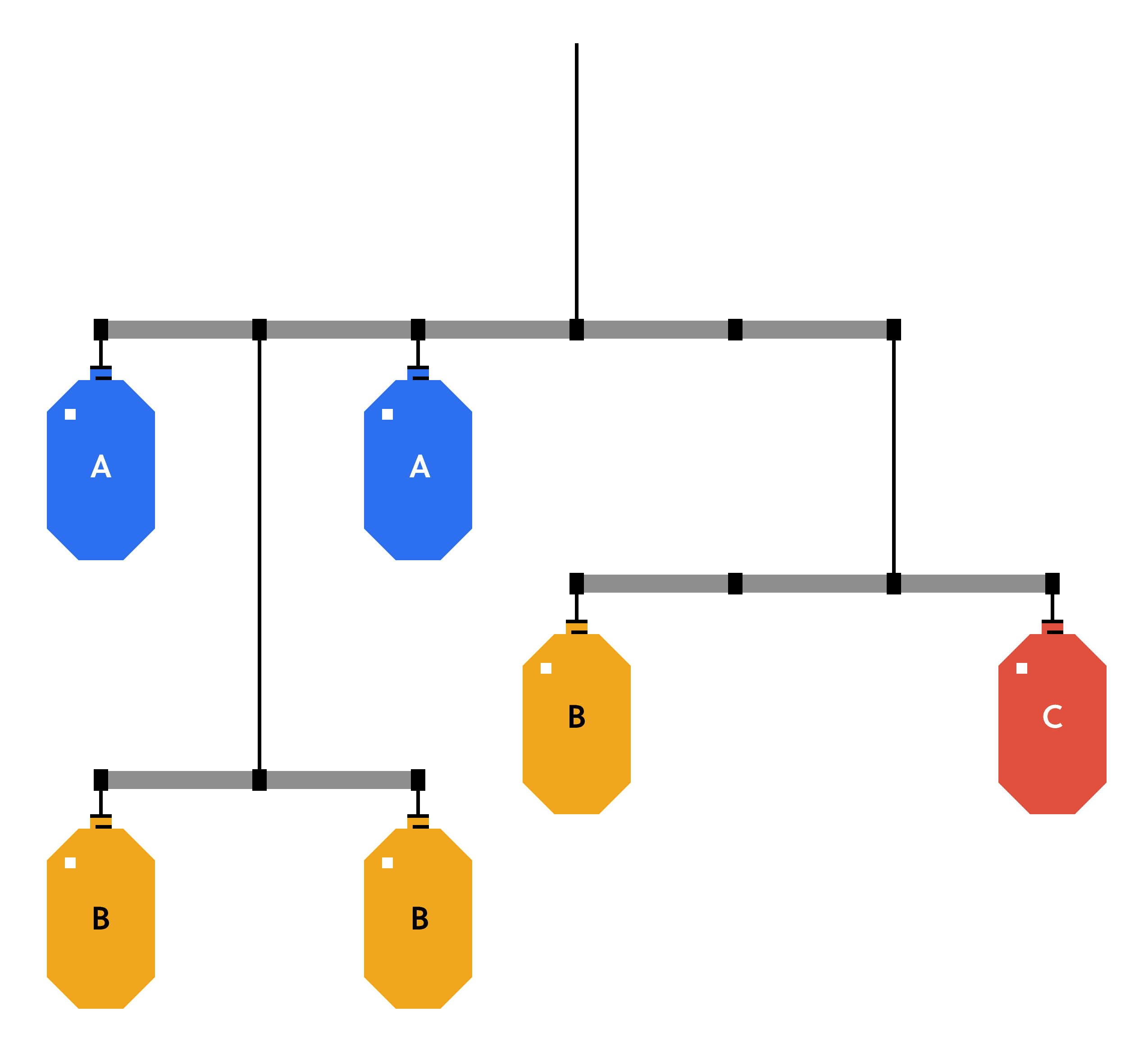## 订单的装饰品

${对齐}\ \开始文本{厘米}& = \压裂{2 \ times0 + 1 \ \ frac35 D + 1 \乘以D}{2 + 1 + 1} \ \ & = \压裂{2}{5}\ \ & = 0.4 D \{对齐}结束$$\{对齐}开始0 & = \压裂{6 \ * \离开(2 l \右)+ 4 x}{6 + 4} \ \ & = \压裂{-12 l + 4 x} {10} \ \ \ Rightarrow x = 3 l . \{对齐}结束$

# 今天的挑战×